Open in App
Not now

# Insertion in a B+ tree

• Difficulty Level : Hard
• Last Updated : 06 Dec, 2022

Prerequisite: Introduction of B+ trees
In this article, we will discuss that how to insert a node in B+ Tree. During insertion following properties of B+ Tree must be followed:

• Each node except root can have a maximum of M children and at least ceil(M/2) children.
• Each node can contain a maximum of M – 1 keys and a minimum of ceil(M/2) – 1 keys.
• The root has at least two children and atleast one search key.
• While insertion overflow of the node occurs when it contains more than M – 1 search key values.

Here M is the order of B+ tree.

### Steps for insertion in B+ Tree

1. Every element is inserted into a leaf node. So, go to the appropriate leaf node.
2. Insert the key into the leaf node in increasing order only if there is no overflow. If there is an overflow go ahead with the following steps mentioned below to deal with overflow while maintaining the B+ Tree properties.

### Properties for insertion B+ Tree

Case 1: Overflow in leaf node

1. Split the leaf node into two nodes.
2. First node contains ceil((m-1)/2) values.
3. Second node contains the remaining values.
4. Copy the smallest search key value from second node to the parent node.(Right biased)

Below is the illustration of inserting 8 into B+ Tree of order of 5: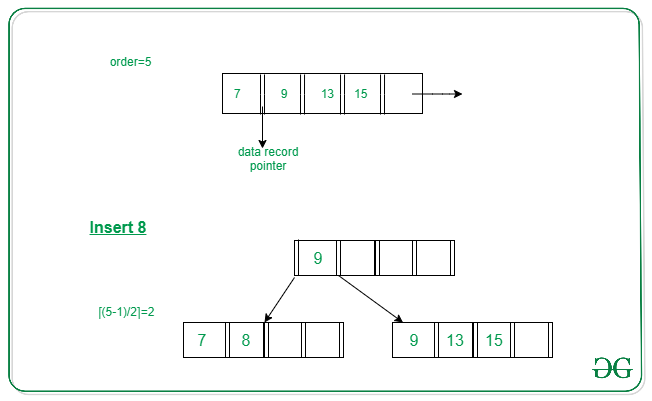Case 2: Overflow in non-leaf node

1. Split the non leaf node into two nodes.
2. First node contains ceil(m/2)-1 values.
3. Move the smallest among remaining to the parent.
4. Second node contains the remaining keys.

Below is the illustration of inserting 15 into B+ Tree of order of 5: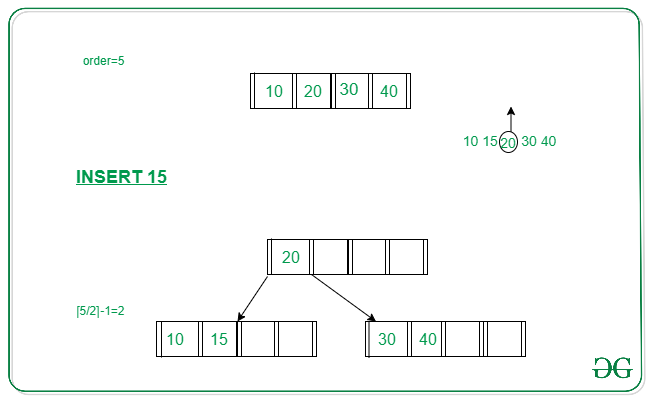## Example to illustrate insertion on a B+ tree

Problem: Insert the following key values 6, 16, 26, 36, 46 on a B+ tree with order = 3.
Solution:
Step 1: The order is 3 so at maximum in a node so there can be only 2 search key values. As insertion happens on a leaf node only in a B+ tree so insert search key value 6 and 16 in increasing order in the node. Below is the illustration of the same: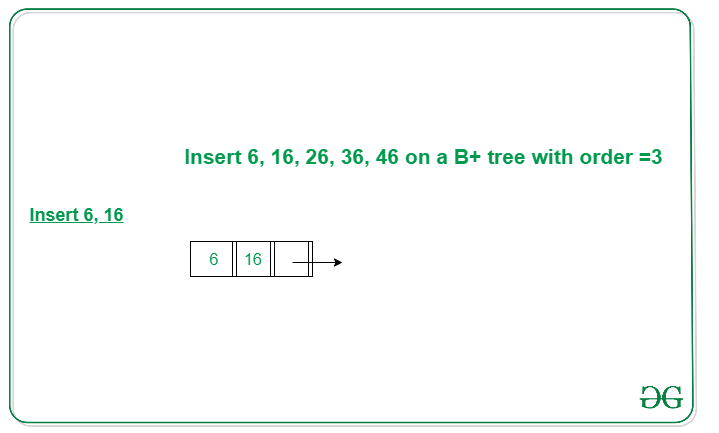Step 2: We cannot insert 26 in the same node as it causes an overflow in the leaf node, We have to split the leaf node according to the rules. First part contains ceil((3-1)/2) values i.e., only 6. The second node contains the remaining values i.e., 16 and 26. Then also copy the smallest search key value from the second node to the parent node i.e., 16 to the parent node. Below is the illustration of the same: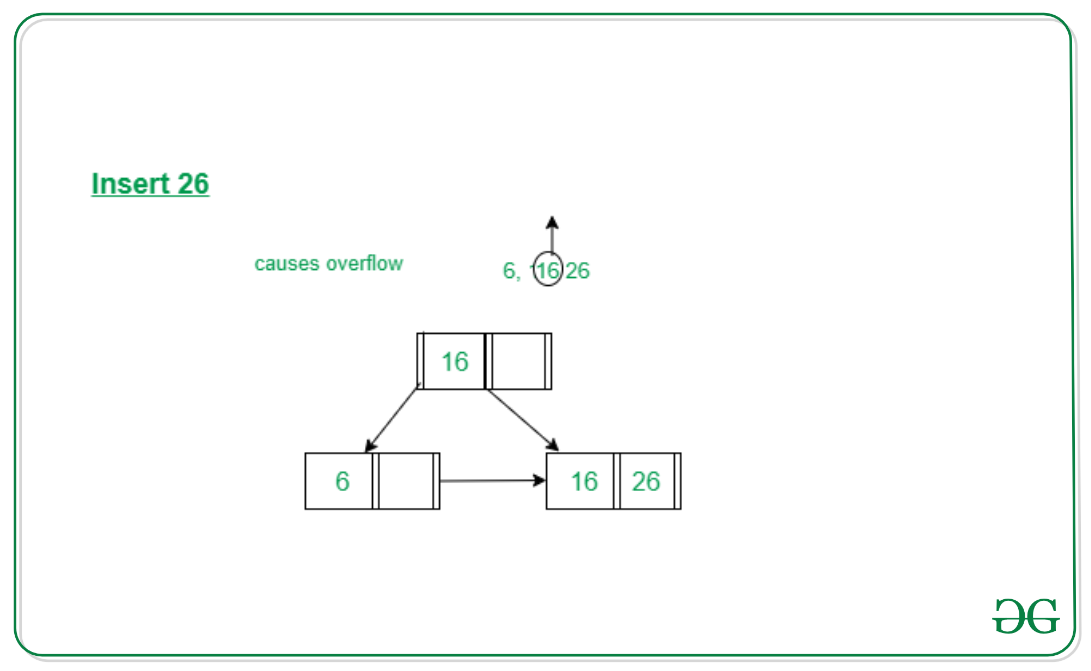Step 3: Now the next value is 36 that is to be inserted after 26 but in that node, it causes an overflow again in that leaf node. Again follow the above steps to split the node. First part contains ceil((3-1)/2) values i.e., only 16. The second node contains the remaining values i.e., 26 and 36. Then also copy the smallest search key value from the second node to the parent node i.e., 26 to the parent node. Below is the illustration of the same:
The illustration is shown in the diagram below.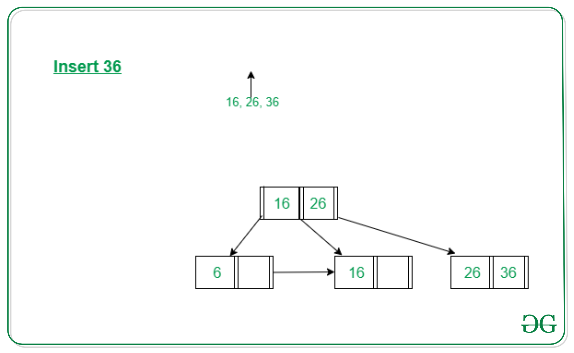Step 4: Now we have to insert 46 which is to be inserted after 36 but it causes an overflow in the leaf node. So we split the node according to the rules. The first part contains 26 and the second part contains 36 and 46 but now we also have to copy 36 to the parent node but it causes overflow as only two search key values can be accommodated in a node. Now follow the steps to deal with overflow in the non-leaf node.
First node contains ceil(3/2)-1 values i.e. ’16’.
Move the smallest among remaining to the parent i.e ’26’ will be the new parent node.
The second node contains the remaining keys i.e ’36’ and the rest of the leaf nodes remain the same. Below is the illustration of the same: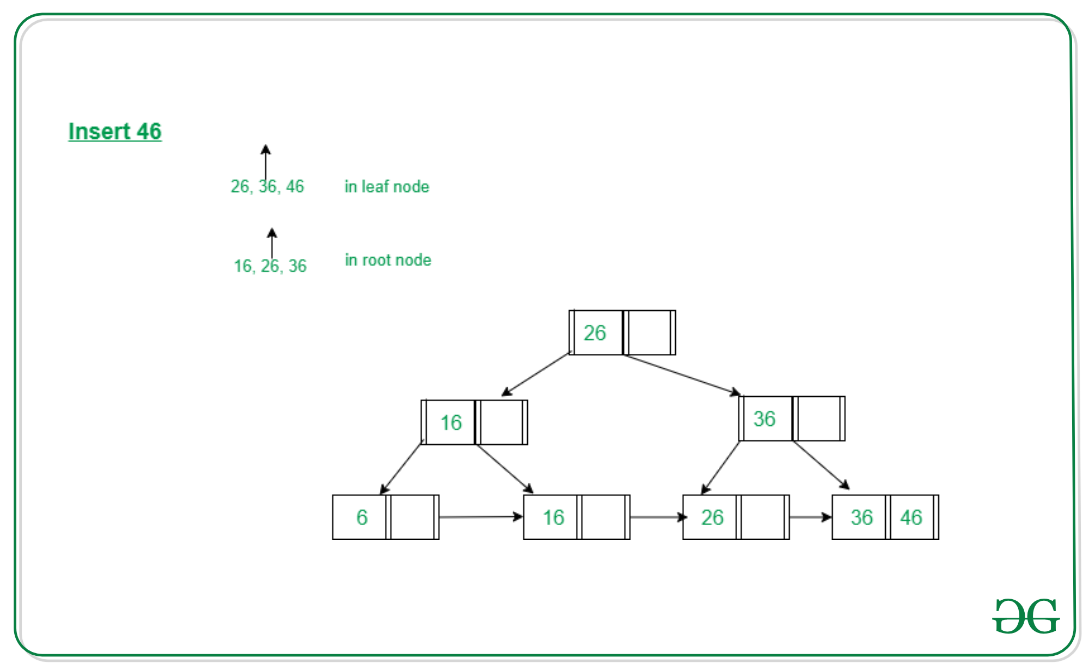Below is the python implementation of B+ tree:

## Python3

 `# Python3 program for implementing B+ Tree`   `import` `math`   `# Node creation` `class` `Node:` `    ``def` `__init__(``self``, order):` `        ``self``.order ``=` `order` `        ``self``.values ``=` `[]` `        ``self``.keys ``=` `[]` `        ``self``.nextKey ``=` `None` `        ``self``.parent ``=` `None` `        ``self``.check_leaf ``=` `False`   `    ``# Insert at the leaf` `    ``def` `insert_at_leaf(``self``, leaf, value, key):` `        ``if` `(``self``.values):` `            ``temp1 ``=` `self``.values` `            ``for` `i ``in` `range``(``len``(temp1)):` `                ``if` `(value ``=``=` `temp1[i]):` `                    ``self``.keys[i].append(key)` `                    ``break` `                ``elif` `(value < temp1[i]):` `                    ``self``.values ``=` `self``.values[:i] ``+` `[value] ``+` `self``.values[i:]` `                    ``self``.keys ``=` `self``.keys[:i] ``+` `[[key]] ``+` `self``.keys[i:]` `                    ``break` `                ``elif` `(i ``+` `1` `=``=` `len``(temp1)):` `                    ``self``.values.append(value)` `                    ``self``.keys.append([key])` `                    ``break` `        ``else``:` `            ``self``.values ``=` `[value]` `            ``self``.keys ``=` `[[key]]`     `# B plus tree` `class` `BplusTree:` `    ``def` `__init__(``self``, order):` `        ``self``.root ``=` `Node(order)` `        ``self``.root.check_leaf ``=` `True`   `    ``# Insert operation` `    ``def` `insert(``self``, value, key):` `        ``value ``=` `str``(value)` `        ``old_node ``=` `self``.search(value)` `        ``old_node.insert_at_leaf(old_node, value, key)`   `        ``if` `(``len``(old_node.values) ``=``=` `old_node.order):` `            ``node1 ``=` `Node(old_node.order)` `            ``node1.check_leaf ``=` `True` `            ``node1.parent ``=` `old_node.parent` `            ``mid ``=` `int``(math.ceil(old_node.order ``/` `2``)) ``-` `1` `            ``node1.values ``=` `old_node.values[mid ``+` `1``:]` `            ``node1.keys ``=` `old_node.keys[mid ``+` `1``:]` `            ``node1.nextKey ``=` `old_node.nextKey` `            ``old_node.values ``=` `old_node.values[:mid ``+` `1``]` `            ``old_node.keys ``=` `old_node.keys[:mid ``+` `1``]` `            ``old_node.nextKey ``=` `node1` `            ``self``.insert_in_parent(old_node, node1.values[``0``], node1)`   `    ``# Search operation for different operations` `    ``def` `search(``self``, value):` `        ``current_node ``=` `self``.root` `        ``while``(current_node.check_leaf ``=``=` `False``):` `            ``temp2 ``=` `current_node.values` `            ``for` `i ``in` `range``(``len``(temp2)):` `                ``if` `(value ``=``=` `temp2[i]):` `                    ``current_node ``=` `current_node.keys[i ``+` `1``]` `                    ``break` `                ``elif` `(value < temp2[i]):` `                    ``current_node ``=` `current_node.keys[i]` `                    ``break` `                ``elif` `(i ``+` `1` `=``=` `len``(current_node.values)):` `                    ``current_node ``=` `current_node.keys[i ``+` `1``]` `                    ``break` `        ``return` `current_node`   `    ``# Find the node` `    ``def` `find(``self``, value, key):` `        ``l ``=` `self``.search(value)` `        ``for` `i, item ``in` `enumerate``(l.values):` `            ``if` `item ``=``=` `value:` `                ``if` `key ``in` `l.keys[i]:` `                    ``return` `True` `                ``else``:` `                    ``return` `False` `        ``return` `False`   `    ``# Inserting at the parent` `    ``def` `insert_in_parent(``self``, n, value, ndash):` `        ``if` `(``self``.root ``=``=` `n):` `            ``rootNode ``=` `Node(n.order)` `            ``rootNode.values ``=` `[value]` `            ``rootNode.keys ``=` `[n, ndash]` `            ``self``.root ``=` `rootNode` `            ``n.parent ``=` `rootNode` `            ``ndash.parent ``=` `rootNode` `            ``return`   `        ``parentNode ``=` `n.parent` `        ``temp3 ``=` `parentNode.keys` `        ``for` `i ``in` `range``(``len``(temp3)):` `            ``if` `(temp3[i] ``=``=` `n):` `                ``parentNode.values ``=` `parentNode.values[:i] ``+` `\` `                    ``[value] ``+` `parentNode.values[i:]` `                ``parentNode.keys ``=` `parentNode.keys[:i ``+` `                                                  ``1``] ``+` `[ndash] ``+` `parentNode.keys[i ``+` `1``:]` `                ``if` `(``len``(parentNode.keys) > parentNode.order):` `                    ``parentdash ``=` `Node(parentNode.order)` `                    ``parentdash.parent ``=` `parentNode.parent` `                    ``mid ``=` `int``(math.ceil(parentNode.order ``/` `2``)) ``-` `1` `                    ``parentdash.values ``=` `parentNode.values[mid ``+` `1``:]` `                    ``parentdash.keys ``=` `parentNode.keys[mid ``+` `1``:]` `                    ``value_ ``=` `parentNode.values[mid]` `                    ``if` `(mid ``=``=` `0``):` `                        ``parentNode.values ``=` `parentNode.values[:mid ``+` `1``]` `                    ``else``:` `                        ``parentNode.values ``=` `parentNode.values[:mid]` `                    ``parentNode.keys ``=` `parentNode.keys[:mid ``+` `1``]` `                    ``for` `j ``in` `parentNode.keys:` `                        ``j.parent ``=` `parentNode` `                    ``for` `j ``in` `parentdash.keys:` `                        ``j.parent ``=` `parentdash` `                    ``self``.insert_in_parent(parentNode, value_, parentdash)`   `# Print the tree` `def` `printTree(tree):` `    ``lst ``=` `[tree.root]` `    ``level ``=` `[``0``]` `    ``leaf ``=` `None` `    ``flag ``=` `0` `    ``lev_leaf ``=` `0`   `    ``node1 ``=` `Node(``str``(level[``0``]) ``+` `str``(tree.root.values))`   `    ``while` `(``len``(lst) !``=` `0``):` `        ``x ``=` `lst.pop(``0``)` `        ``lev ``=` `level.pop(``0``)` `        ``if` `(x.check_leaf ``=``=` `False``):` `            ``for` `i, item ``in` `enumerate``(x.keys):` `                ``print``(item.values)` `        ``else``:` `            ``for` `i, item ``in` `enumerate``(x.keys):` `                ``print``(item.values)` `            ``if` `(flag ``=``=` `0``):` `                ``lev_leaf ``=` `lev` `                ``leaf ``=` `x` `                ``flag ``=` `1`     `record_len ``=` `3` `bplustree ``=` `BplusTree(record_len)` `bplustree.insert(``'5'``, ``'33'``)` `bplustree.insert(``'15'``, ``'21'``)` `bplustree.insert(``'25'``, ``'31'``)` `bplustree.insert(``'35'``, ``'41'``)` `bplustree.insert(``'45'``, ``'10'``)`   `printTree(bplustree)`   `if``(bplustree.find(``'5'``, ``'34'``)):` `    ``print``(``"Found"``)` `else``:` `    ``print``(``"Not found"``)`

Output

```['15', '25']
['35', '45']
['5']
```

Time complexity: O(log n)
Auxiliary Space: O(log n)

Below is the C++ implementation B+ tree:

## C++

 `#include ` `using` `namespace` `std;` `typedef` `long` `long` `ll;` `typedef` `unsigned ``long` `long` `ull;` `#define pb push_back`   `int` `bucketSize = 3;`   `// Create 2 classes, one for node and one for tree;`   `class` `node {` `public``:` `    ``bool` `isLeaf;` `    ``node** ptr;` `    ``int` `*key, size;` `    ``node();` `};` `node::node()` `{` `    ``key = ``new` `int``[bucketSize];` `    ``ptr = ``new` `node*[bucketSize + 1];` `}` `class` `Btree {` `public``:` `    ``// Root of tree stored here;` `    ``node* root;` `    ``Btree();` `    ``void` `deleteNode(``int``);`   `    ``int` `search(``int``);` `    ``void` `display(node*);` `    ``void` `insert(``int``);` `    ``node* findParent(node*, node*);` `    ``node* getRoot();` `    ``void` `shiftLevel(``int``, node*, node*);` `};`   `node* Btree::getRoot() { ``return` `root; }` `Btree::Btree() { root = NULL; }`   `void` `Btree::insert(``int` `x)` `{` `    ``if` `(root == NULL) {` `        ``root = ``new` `node;` `        ``root->key = x;` `        ``root->isLeaf = ``true``;` `        ``root->size = 1;` `    ``}`   `    ``else` `{` `        ``node* current = root;` `        ``node* parent;`   `        ``while` `(current->isLeaf == ``false``) {` `            ``parent = current;`   `            ``for` `(``int` `i = 0; i < current->size; i++) {` `                ``if` `(x < current->key[i]) {` `                    ``current = current->ptr[i];` `                    ``break``;` `                ``}`   `                ``if` `(i == current->size - 1) {` `                    ``current = current->ptr[i + 1];` `                    ``break``;` `                ``}` `            ``}` `        ``}`   `        ``// now we have reached leaf;` `        ``if` `(current->size` `            ``< bucketSize) { ``// if the node to be inserted is` `                            ``// not filled` `            ``int` `i = 0;`   `            ``// Traverse btree` `            ``while` `(x > current->key[i] && i < current->size)` `                ``// goto pt where needs to be inserted.` `                ``i++;`   `            ``for` `(``int` `j = current->size; j > i; j--)` `                ``// adjust and insert element;` `                ``current->key[j] = current->key[j - 1];`   `            ``current->key[i] = x;`   `            ``// size should be increased by 1` `            ``current->size++;`   `            ``current->ptr[current->size]` `                ``= current->ptr[current->size - 1];` `            ``current->ptr[current->size - 1] = NULL;` `        ``}`   `        ``// if block does not have enough space;` `        ``else` `{` `            ``node* newLeaf = ``new` `node;` `            ``int` `tempNode[bucketSize + 1];`   `            ``for` `(``int` `i = 0; i < bucketSize; i++)` `                ``// all elements of this block stored` `                ``tempNode[i] = current->key[i];` `            ``int` `i = 0, j;`   `            ``// find the right posn of num to be inserted` `            ``while` `(x > tempNode[i] && i < bucketSize)` `                ``i++;`   `            ``for` `(``int` `j = bucketSize + 1; j > i; j--)` `                ``tempNode[j] = tempNode[j - 1];` `            ``tempNode[i] = x;` `            ``// inserted element in its rightful position;`   `            ``newLeaf->isLeaf = ``true``;` `            ``current->size = (bucketSize + 1) / 2;` `            ``newLeaf->size` `                ``= (bucketSize + 1)` `                  ``- (bucketSize + 1)` `                        ``/ 2; ``// now rearrangement begins!`   `            ``current->ptr[current->size] = newLeaf;` `            ``newLeaf->ptr[newLeaf->size]` `                ``= current->ptr[bucketSize];`   `            ``current->ptr[newLeaf->size]` `                ``= current->ptr[bucketSize];` `            ``current->ptr[bucketSize] = NULL;`   `            ``for` `(``int` `i = 0; i < current->size; i++)` `                ``current->key[i] = tempNode[i];`   `            ``for` `(``int` `i = 0, j = current->size;` `                 ``i < newLeaf->size; i++, j++)` `                ``newLeaf->key[i] = tempNode[j];`   `            ``// if this is root, then fine,` `            ``// else we need to increase the height of tree;` `            ``if` `(current == root) {` `                ``node* newRoot = ``new` `node;` `                ``newRoot->key = newLeaf->key;` `                ``newRoot->ptr = current;` `                ``newRoot->ptr = newLeaf;` `                ``newRoot->isLeaf = ``false``;` `                ``newRoot->size = 1;` `                ``root = newRoot;` `            ``}` `            ``else` `                ``shiftLevel(` `                    ``newLeaf->key, parent,` `                    ``newLeaf); ``// parent->original root` `        ``}` `    ``}` `}`   `void` `Btree::shiftLevel(``int` `x, node* current, node* child)` `{ ``// insert or create an internal node;` `    ``if` `(current->size` `        ``< bucketSize) { ``// if can fit in this level, do that` `        ``int` `i = 0;` `        ``while` `(x > current->key[i] && i < current->size)` `            ``i++;` `        ``for` `(``int` `j = current->size; j > i; j--)` `            ``current->key[j] = current->key[j - 1];`   `        ``for` `(``int` `j = current->size + 1; j > i + 1; j--)` `            ``current->ptr[j] = current->ptr[j - 1];`   `        ``current->key[i] = x;` `        ``current->size++;` `        ``current->ptr[i + 1] = child;` `    ``}`   `    ``// shift up` `    ``else` `{` `        ``node* newInternal = ``new` `node;` `        ``int` `tempKey[bucketSize + 1];` `        ``node* tempPtr[bucketSize + 2];`   `        ``for` `(``int` `i = 0; i < bucketSize; i++)` `            ``tempKey[i] = current->key[i];`   `        ``for` `(``int` `i = 0; i < bucketSize + 1; i++)` `            ``tempPtr[i] = current->ptr[i];`   `        ``int` `i = 0, j;` `        ``while` `(x > tempKey[i] && i < bucketSize)` `            ``i++;`   `        ``for` `(``int` `j = bucketSize + 1; j > i; j--)` `            ``tempKey[j] = tempKey[j - 1];`   `        ``tempKey[i] = x;` `        ``for` `(``int` `j = bucketSize + 2; j > i + 1; j--)` `            ``tempPtr[j] = tempPtr[j - 1];`   `        ``tempPtr[i + 1] = child;` `        ``newInternal->isLeaf = ``false``;` `        ``current->size = (bucketSize + 1) / 2;`   `        ``newInternal->size` `            ``= bucketSize - (bucketSize + 1) / 2;`   `        ``for` `(``int` `i = 0, j = current->size + 1;` `             ``i < newInternal->size; i++, j++)` `            ``newInternal->key[i] = tempKey[j];`   `        ``for` `(``int` `i = 0, j = current->size + 1;` `             ``i < newInternal->size + 1; i++, j++)` `            ``newInternal->ptr[i] = tempPtr[j];`   `        ``if` `(current == root) {` `            ``node* newRoot = ``new` `node;` `            ``newRoot->key = current->key[current->size];` `            ``newRoot->ptr = current;` `            ``newRoot->ptr = newInternal;` `            ``newRoot->isLeaf = ``false``;` `            ``newRoot->size = 1;` `            ``root = newRoot;` `        ``}`   `        ``else` `            ``shiftLevel(current->key[current->size],` `                       ``findParent(root, current),` `                       ``newInternal);` `    ``}` `}` `int` `Btree::search(``int` `x)` `{` `    ``if` `(root == NULL)` `        ``return` `-1;`   `    ``else` `{` `        ``node* current = root;` `        ``while` `(current->isLeaf == ``false``) {` `            ``for` `(``int` `i = 0; i < current->size; i++) {` `                ``if` `(x < current->key[i]) {` `                    ``current = current->ptr[i];` `                    ``break``;` `                ``}`   `                ``if` `(i == current->size - 1) {` `                    ``current = current->ptr[i + 1];` `                    ``break``;` `                ``}` `            ``}` `        ``}`   `        ``for` `(``int` `i = 0; i < current->size; i++) {` `            ``if` `(current->key[i] == x) {` `                ``// cout<<"Key found "< q;` `    ``q.push(current);` `    ``while` `(!q.empty()) {` `        ``int` `l;` `        ``l = q.size();`   `        ``for` `(``int` `i = 0; i < l; i++) {` `            ``node* tNode = q.front();` `            ``q.pop();`   `            ``for` `(``int` `j = 0; j < tNode->size; j++)` `                ``if` `(tNode != NULL)` `                    ``cout << tNode->key[j] << ``" "``;`   `            ``for` `(``int` `j = 0; j < tNode->size + 1; j++)` `                ``if` `(tNode->ptr[j] != NULL)` `                    ``q.push(tNode->ptr[j]);`   `            ``cout << ``"\t"``;` `        ``}` `        ``cout << endl;` `    ``}` `}`   `node* Btree::findParent(node* current, node* child)` `{` `    ``node* parent;` `    ``if` `(current->isLeaf || (current->ptr)->isLeaf)` `        ``return` `NULL;`   `    ``for` `(``int` `i = 0; i < current->size + 1; i++) {` `        ``if` `(current->ptr[i] == child) {` `            ``parent = current;` `            ``return` `parent;` `        ``}` `        ``else` `{` `            ``parent = findParent(current->ptr[i], child);` `            ``if` `(parent != NULL)` `                ``return` `parent;` `        ``}` `    ``}` `    ``return` `parent;` `}`   `signed` `main()` `{` `    ``ios_base::sync_with_stdio(``false``);` `    ``Btree node;` `    ``cout << ``"The size of bucket is "` `<< bucketSize << ``"! "` `         ``<< endl;`   `    ``node.insert(1);` `    ``node.insert(2);` `    ``node.insert(3);` `    ``node.display(node.getRoot());` `    ``node.insert(4);` `    ``node.insert(5);` `    ``node.display(node.getRoot());`   `    ``return` `0;` `}`

Output

```The size of bucket is 3!
1 2 3
3
1 2     3 4 5

```

Time Complexity:

• Insertion: O(log (h*bucketSize)), where h is height of the tree, and bucketSize denotes the number of elements that can be stored in a single bucket.
• Deletion: O(log (h*bucketSize))

Auxiliary Space: O(n), n-> number of elements in the tree.

My Personal Notes arrow_drop_up
Related Articles